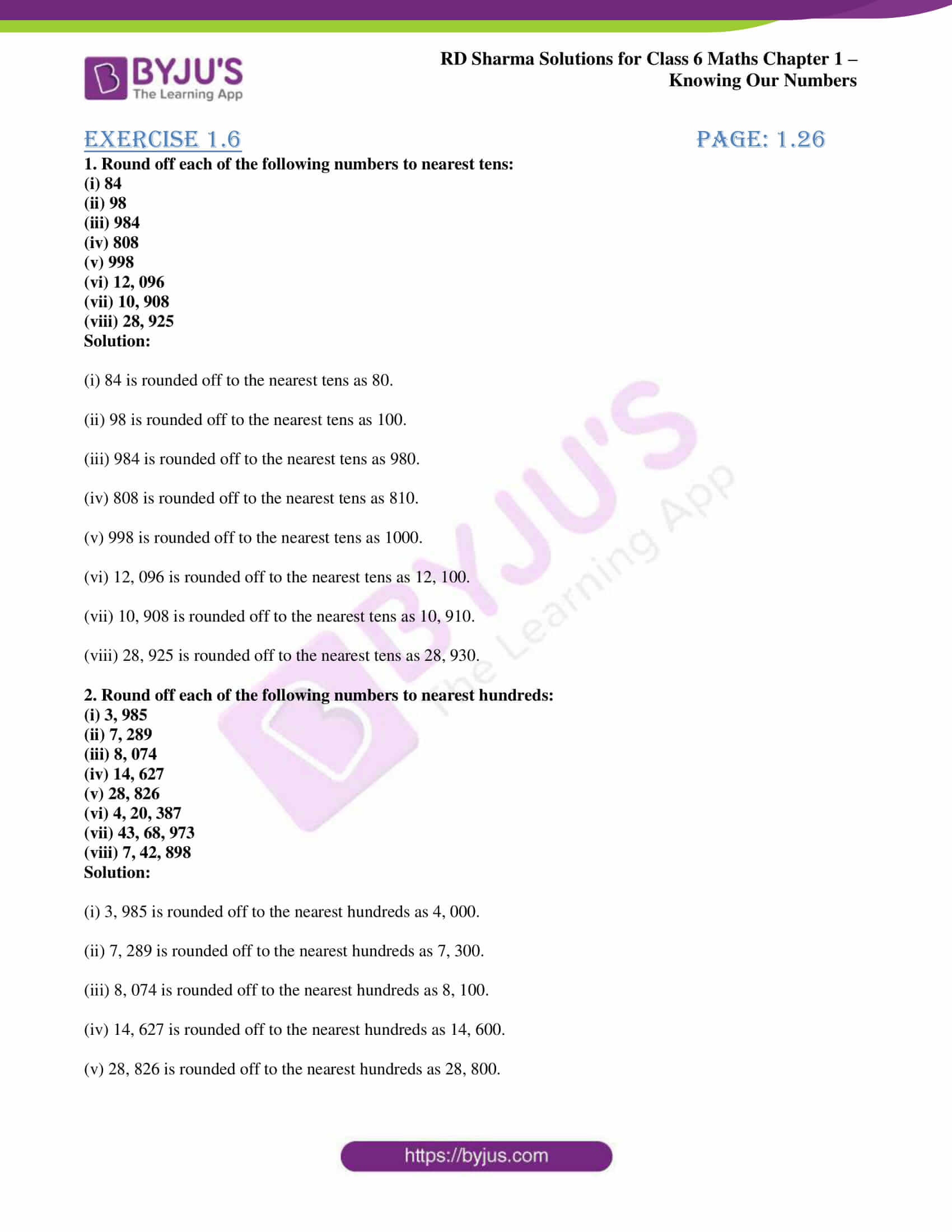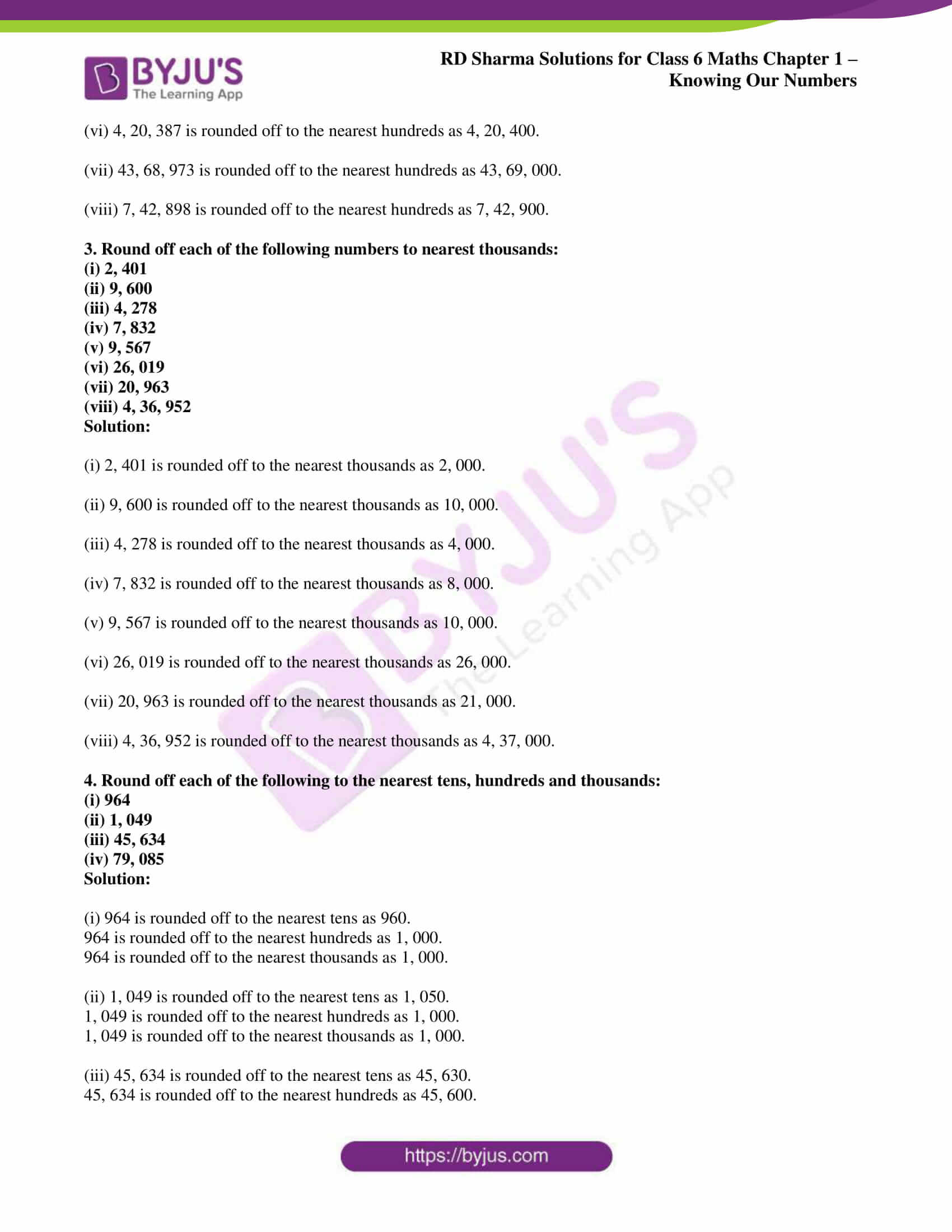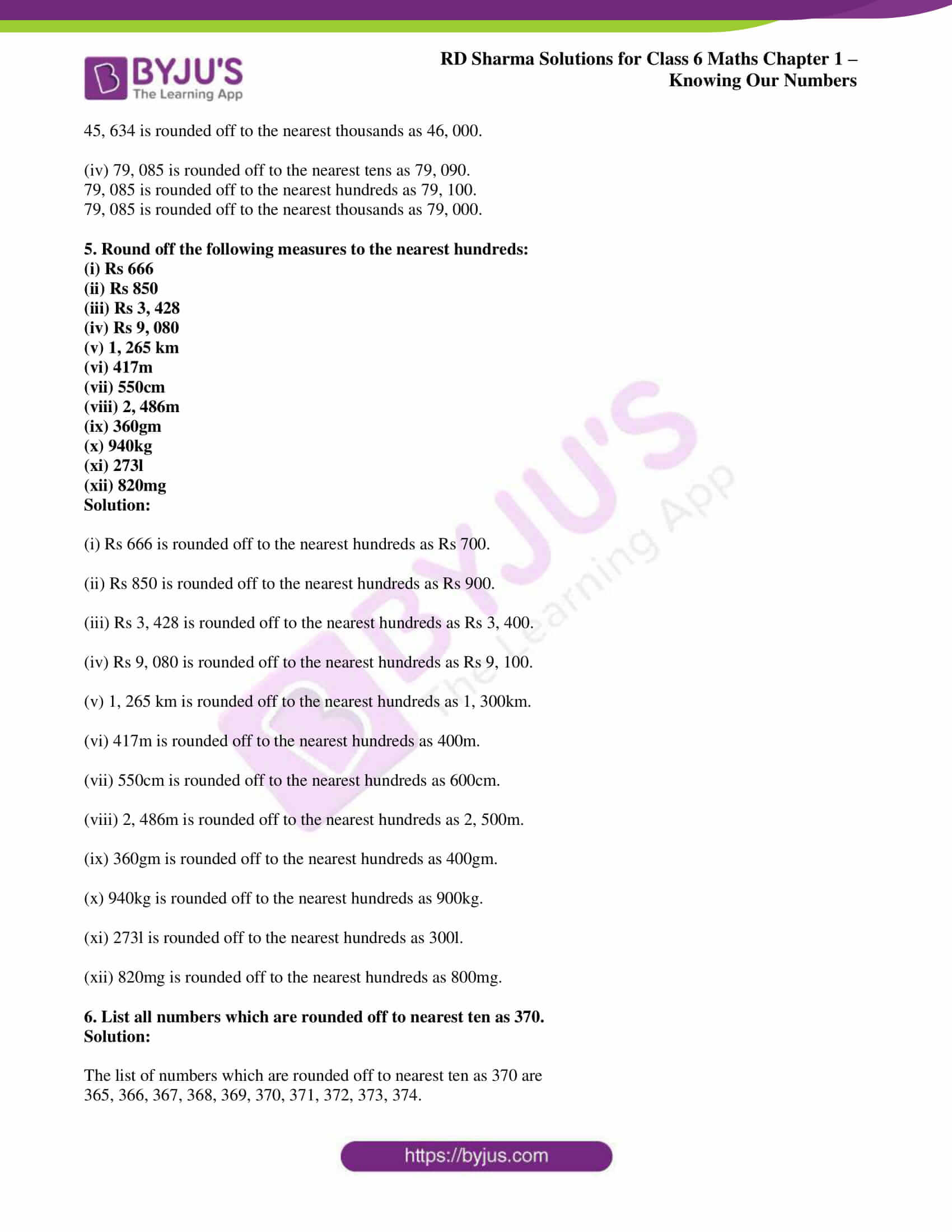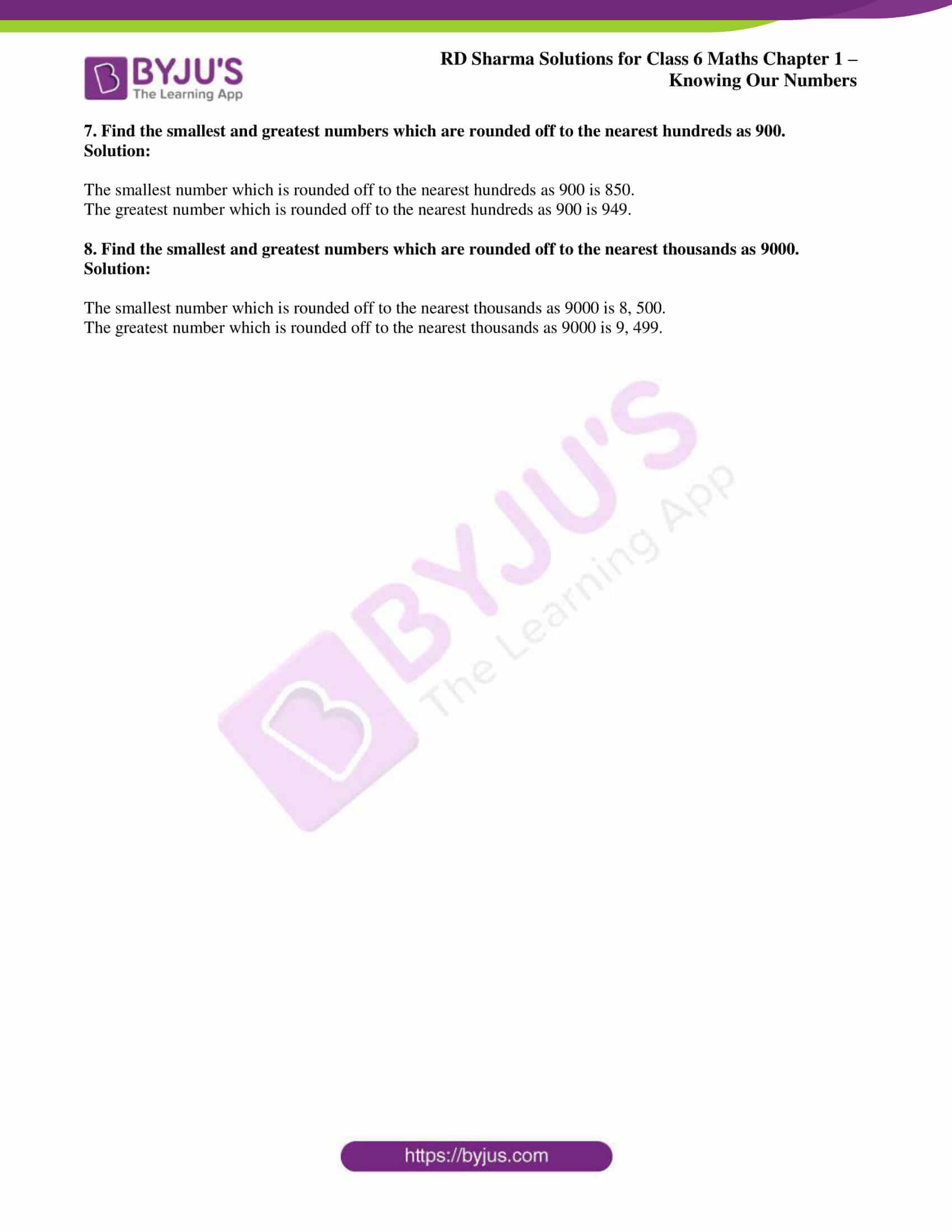# RD Sharma Solutions for Class 6 Maths Chapter 1: Knowing Our Numbers Exercise 1.6

## RD Sharma Solutions for Class 6 Maths Exercise 1.6 PDF

The experts at BYJU’S provide detailed answers to exercise wise problems based on RD Sharma textbook. The students while solving problems can use the PDF in order to get a good score in the exam. Helping the students to become clear with the concepts which are covered in each exercise is the main aim of creating solutions. The PDF of solutions can be downloaded easily by the students based on the chapter or exercise required to practice. The students by solving problems of each exercise can analyse the areas which require improvement. RD Sharma Solutions for Class 6 Maths Chapter 1 Knowing Our Numbers Exercise 1.6 are provided here.

## RD Sharma Solutions for Class 6 Maths Chapter 1: Knowing Our Numbers Exercise 1.6 Download PDF## Access answers to Maths RD Sharma Solutions for Class 6 Chapter 1: Knowing Our Numbers Exercise 1.6

#### Exercise 1.6 page: 1.26

1. Round off each of the following numbers to nearest tens:

(i) 84

(ii) 98

(iii) 984

(iv) 808

(v) 998

(vi) 12, 096

(vii) 10, 908

(viii) 28, 925

Solution:

(i) 84 is rounded off to the nearest tens as 80.

(ii) 98 is rounded off to the nearest tens as 100.

(iii) 984 is rounded off to the nearest tens as 980.

(iv) 808 is rounded off to the nearest tens as 810.

(v) 998 is rounded off to the nearest tens as 1000.

(vi) 12, 096 is rounded off to the nearest tens as 12, 100.

(vii) 10, 908 is rounded off to the nearest tens as 10, 910.

(viii) 28, 925 is rounded off to the nearest tens as 28, 930.

2. Round off each of the following numbers to nearest hundreds:

(i) 3, 985

(ii) 7, 289

(iii) 8, 074

(iv) 14, 627

(v) 28, 826

(vi) 4, 20, 387

(vii) 43, 68, 973

(viii) 7, 42, 898

Solution:

(i) 3, 985 is rounded off to the nearest hundreds as 4, 000.

(ii) 7, 289 is rounded off to the nearest hundreds as 7, 300.

(iii) 8, 074 is rounded off to the nearest hundreds as 8, 100.

(iv) 14, 627 is rounded off to the nearest hundreds as 14, 600.

(v) 28, 826 is rounded off to the nearest hundreds as 28, 800.

(vi) 4, 20, 387 is rounded off to the nearest hundreds as 4, 20, 400.

(vii) 43, 68, 973 is rounded off to the nearest hundreds as 43, 69, 000.

(viii) 7, 42, 898 is rounded off to the nearest hundreds as 7, 42, 900.

3. Round off each of the following numbers to nearest thousands:

(i) 2, 401

(ii) 9, 600

(iii) 4, 278

(iv) 7, 832

(v) 9, 567

(vi) 26, 019

(vii) 20, 963

(viii) 4, 36, 952

Solution:

(i) 2, 401 is rounded off to the nearest thousands as 2, 000.

(ii) 9, 600 is rounded off to the nearest thousands as 10, 000.

(iii) 4, 278 is rounded off to the nearest thousands as 4, 000.

(iv) 7, 832 is rounded off to the nearest thousands as 8, 000.

(v) 9, 567 is rounded off to the nearest thousands as 10, 000.

(vi) 26, 019 is rounded off to the nearest thousands as 26, 000.

(vii) 20, 963 is rounded off to the nearest thousands as 21, 000.

(viii) 4, 36, 952 is rounded off to the nearest thousands as 4, 37, 000.

4. Round off each of the following to the nearest tens, hundreds and thousands:

(i) 964

(ii) 1, 049

(iii) 45, 634

(iv) 79, 085

Solution:

(i) 964 is rounded off to the nearest tens as 960.

964 is rounded off to the nearest hundreds as 1, 000.

964 is rounded off to the nearest thousands as 1, 000.

(ii) 1, 049 is rounded off to the nearest tens as 1, 050.

1, 049 is rounded off to the nearest hundreds as 1, 000.

1, 049 is rounded off to the nearest thousands as 1, 000.

(iii) 45, 634 is rounded off to the nearest tens as 45, 630.

45, 634 is rounded off to the nearest hundreds as 45, 600.

45, 634 is rounded off to the nearest thousands as 46, 000.

(iv) 79, 085 is rounded off to the nearest tens as 79, 090.

79, 085 is rounded off to the nearest hundreds as 79, 100.

79, 085 is rounded off to the nearest thousands as 79, 000.

5. Round off the following measures to the nearest hundreds:

(i) Rs 666

(ii) Rs 850

(iii) Rs 3, 428

(iv) Rs 9, 080

(v) 1, 265 km

(vi) 417m

(vii) 550cm

(viii) 2, 486m

(ix) 360gm

(x) 940kg

(xi) 273l

(xii) 820mg

Solution:

(i) Rs 666 is rounded off to the nearest hundreds as Rs 700.

(ii) Rs 850 is rounded off to the nearest hundreds as Rs 900.

(iii) Rs 3, 428 is rounded off to the nearest hundreds as Rs 3, 400.

(iv) Rs 9, 080 is rounded off to the nearest hundreds as Rs 9, 100.

(v) 1, 265 km is rounded off to the nearest hundreds as 1, 300km.

(vi) 417m is rounded off to the nearest hundreds as 400m.

(vii) 550cm is rounded off to the nearest hundreds as 600cm.

(viii) 2, 486m is rounded off to the nearest hundreds as 2, 500m.

(ix) 360gm is rounded off to the nearest hundreds as 400gm.

(x) 940kg is rounded off to the nearest hundreds as 900kg.

(xi) 273l is rounded off to the nearest hundreds as 300l.

(xii) 820mg is rounded off to the nearest hundreds as 800mg.

6. List all numbers which are rounded off to nearest ten as 370.

Solution:

The list of numbers which are rounded off to nearest ten as 370 are

365, 366, 367, 368, 369, 370, 371, 372, 373, 374.

7. Find the smallest and greatest numbers which are rounded off to the nearest hundreds as 900.

Solution:

The smallest number which is rounded off to the nearest hundreds as 900 is 850.

The greatest number which is rounded off to the nearest hundreds as 900 is 949.

8. Find the smallest and greatest numbers which are rounded off to the nearest thousands as 9000.

Solution:

The smallest number which is rounded off to the nearest thousands as 9000 is 8, 500.

The greatest number which is rounded off to the nearest thousands as 9000 is 9, 499.

### RD Sharma Solutions Class 6 Maths Chapter 1 – Knowing Our Numbers Exercise 1.6

RD Sharma Solutions Class 6 Maths Chapter 1 Knowing Our Numbers Exercise 1.6 tells us about the methods of rounding off given set of numbers to the nearest tens, hundreds and thousands.

### Key features of RD Sharma Solutions for Class 6 Maths Chapter 1: Knowing Our Numbers Exercise 1.6

• The solutions are created based on latest CBSE syllabus and exam pattern for Class 6.
• PDF contains solutions to all the problems which are covered in each exercise of RD Sharma textbook.
• The students gain a better knowledge about the topics which are explained under each exercise.
• Self evaluation is improved among students by solving huge number of examples and problems.